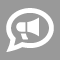#Blogs

## Logarithms Blogs

Hey guys,     After working with high school and college students in algebra 1 & 2,  i came across quite a few students who found logarithms hard to work with . Most of the  students failed to retain some important log based rules which were essential to be effective at problem solving.   Here's a list of a few rules :   logab = x could be written as  ax = b. Let me work a couple of examples to show how this rule works   log39 = 2  By using the rule that i talked about above can be written as 3^2 = 9 where a=3, x=2 and b = 9.      loga10 - loga5  can be rewritten in the form loga(10/5)  which is the same as loga2. As long as the bases are equal to one another we can divide when there is a subtraction taking place using log.   When it comes to addition we need to add logarithms that have the same base.     Also... read more

One of the main complaints that students have when struggling with their math homework is that they don't understand why they need to learn this in the first place.  After all, how often do we actually use calculus or trigonometry in our daily lives?   I always make an effort to correct this false assumption in my students.  Everything that we learn in math connects to reality in often unexpected ways.  For this reason, I like to find out what it is that interests my student, or what their career goals are, so that I may show them how the math connects.   Take the example of logarithms.  For the student with an ear for music, I can explain how logarithmic scales describe the relationships between musical tones, and true understanding of musical theory requires an understanding of this field of math.  For the student who plans to go into the medical field, logarithms can be used to help model the levels of medications in a patient's... read more

This journey is heavily inspired by the youtube mathematician Vi Hart, whose videos describing mathematical concepts through doodling in a notebook were the inspiration for much of my mathematical journeys series. I'll put a link to her video on this topic at the end of the journey, and I highly encourage everyone to go check her out. Let's talk exponents. But to do that, first we should talk about multiplication. Multiplication is a shortcut for adding a bunch of the same number together. If I gave you: 5 + 5 + 5 + 5 + 5 + 5 = ? You could just add them normally, treating each of those 5's as a size-5 step along the number line. But since each of these addition steps is the same size, a faster way to figure out the result would be to determine two things: the size of the step, and how many steps we have. Then we can multiply the size of step (in this case, 5) by the number of steps. In this case, we have a total of 6 size-5 steps,... read more

Thanks, Vihart of YouTube!   In this video, elementary algebra is built up from counting to positive numbers to negative numbers to multiplication and division to exponents and logarithms. All these concepts are tied together under the common theme of "fancy counting." That is, each of these operations is tied directly to the fundamental operation of "+1".   All of this in just over 9 minutes. This is how experts think of algebra. Novices, new to the world of algebra, see the mechanical steps to be taken, the shuffling of symbols, the confusing mass of numbers, letters, and symbols and get lost. Experts think of algebra as chunks of value that are being operated upon by a (relatively) small set of operations, made smaller by lumping an operation with its inverse (addition/subtraction, multiplication/division, exponents/logarithms) and realizing they are two sides of the same coin.   "How I Feel About Logarithms, by...